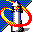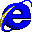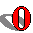## File size

• This script works only in Explorer 4+ on Windows. The Mac versions can calculate the file size of the page, but not of the images. Since this is a developer's script, for once I don't care (too much).
•

When you're almost finished making a page, it's very useful to check how large it is. The standard for an ideal WWW page is about 40K in total (HTML plus images) and it's generally considered wise not to exceed this norm, unless for a very specific reason.

Unfortunately I found out that it's not possible to also calculate the size of included JavaScripts and style sheets. However, generally they're not very large, I suggest adding about 1K per style sheet or JS include to the total.

### Example### The script

This is the script:

```function calculate()
{
if (!document.fileSize)
{
return;
}
var size = (document.fileSize)*1;
var y = document.images;
var imglength = 0;
for (i=0;i<y.length;i++)
{
imglength += (y[i].fileSize)*1;
}
var total = size + imglength;
var writestring = 'File size HTML: ' + size;
writestring += '\nFile size images: ' + imglength;
writestring += '\nTotal file size: ' + total;
if (total > 40000) writestring += "\nFile too large!";
}
```

### Explanation

First of all, if the browser doesn't support fileSize end the function.

```function calculate()
{
if (!document.fileSize)
{
return;
}
```

Calculate the file size of the HTML document, then multiply it by 1 because it is a string and we want a number.

```	var size = (document.fileSize)*1;
```

Calculate the file size of each of the images and make imglength the total.

```	var y = document.images;
var imglength = 0;
for (i=0;i<y.length;i++)
{
imglength += (y[i].fileSize)*1;
}
```

Add HTML and images file sizes.

```	var total = size + imglength;
```

Then create a string with the information and alert it.

```	var writestring = 'File size HTML: ' + size;
writestring += '\nFile size images: ' + imglength;
writestring += '\nTotal file size: ' + total;
if (total > 40000) writestring += "\nFile too large!";